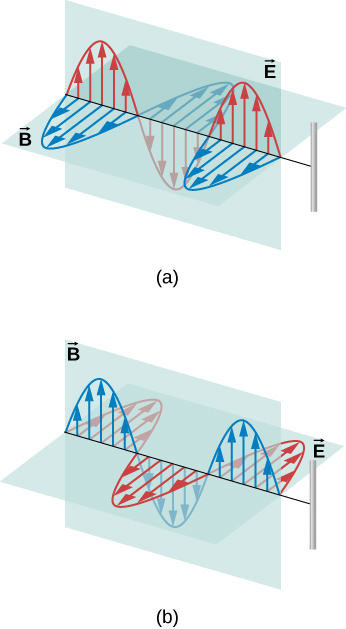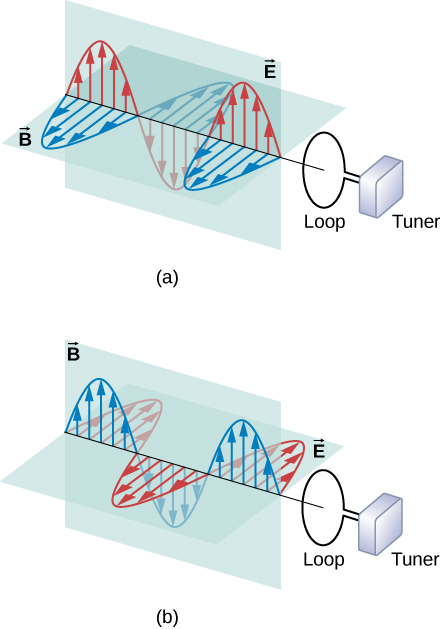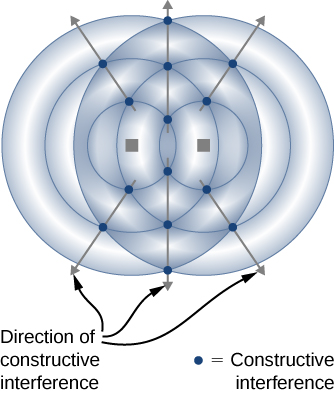# 16.2 Plane electromagnetic waves  (Page 5/5)

 Page 5 / 5

The electric field lines in one plane are shown. The magnetic field is perpendicular to this plane. This radiation field has cylindrical symmetry around the axis of the dipole. Field lines near the dipole are not shown. The pattern is not at all uniform in all directions. The strongest signal is in directions perpendicular to the axis of the antenna, which would be horizontal if the antenna is mounted vertically. There is zero intensity along the axis of the antenna. The fields detected far from the antenna are from the changing electric and magnetic fields inducing each other and traveling as electromagnetic waves. Far from the antenna, the wave fronts, or surfaces of equal phase for the electromagnetic wave, are almost spherical. Even farther from the antenna, the radiation propagates like electromagnetic plane waves.

The electromagnetic waves carry energy away from their source, similar to a sound wave carrying energy away from a standing wave on a guitar string. An antenna for receiving electromagnetic signals works in reverse. Incoming electromagnetic waves induce oscillating currents in the antenna, each at its own frequency. The radio receiver includes a tuner circuit, whose resonant frequency can be adjusted. The tuner responds strongly to the desired frequency but not others, allowing the user to tune to the desired broadcast. Electrical components amplify the signal formed by the moving electrons. The signal is then converted into an audio and/or video format.

Use this simulation to broadcast radio waves. Wiggle the transmitter electron manually or have it oscillate automatically. Display the field as a curve or vectors. The strip chart shows the electron positions at the transmitter and at the receiver.

## Summary

• Maxwell’s equations predict that the directions of the electric and magnetic fields of the wave, and the wave’s direction of propagation, are all mutually perpendicular. The electromagnetic wave is a transverse wave.
• The strengths of the electric and magnetic parts of the wave are related by $c=E\text{/}B,$ which implies that the magnetic field B is very weak relative to the electric field E .
• Accelerating charges create electromagnetic waves (for example, an oscillating current in a wire produces electromagnetic waves with the same frequency as the oscillation).

## Conceptual questions

If the electric field of an electromagnetic wave is oscillating along the z -axis and the magnetic field is oscillating along the x -axis, in what possible direction is the wave traveling?

In which situation shown below will the electromagnetic wave be more successful in inducing a current in the wire? Explain.in (a), because the electric field is parallel to the wire, accelerating the electrons

In which situation shown below will the electromagnetic wave be more successful in inducing a current in the loop? Explain.Under what conditions might wires in a circuit where the current flows in only one direction emit electromagnetic waves?

A steady current in a dc circuit will not produce electromagnetic waves. If the magnitude of the current varies while remaining in the same direction, the wires will emit electromagnetic waves, for example, if the current is turned on or off.

Shown below is the interference pattern of two radio antennas broadcasting the same signal. Explain how this is analogous to the interference pattern for sound produced by two speakers. Could this be used to make a directional antenna system that broadcasts preferentially in certain directions? Explain.## Problems

If the Sun suddenly turned off, we would not know it until its light stopped coming. How long would that be, given that the Sun is $1.496\phantom{\rule{0.2em}{0ex}}×\phantom{\rule{0.2em}{0ex}}{10}^{11}\phantom{\rule{0.2em}{0ex}}\text{m}$ away?

499 s

What is the maximum electric field strength in an electromagnetic wave that has a maximum magnetic field strength of $5.00\phantom{\rule{0.2em}{0ex}}×\phantom{\rule{0.2em}{0ex}}{10}^{-4}\phantom{\rule{0.2em}{0ex}}\text{T}$ (about 10 times Earth’s magnetic field)?

An electromagnetic wave has a frequency of 12 MHz. What is its wavelength in vacuum?

25 m

If electric and magnetic field strengths vary sinusoidally in time at frequency 1.00 GHz, being zero at $t=0$ , then $E={E}_{0}\phantom{\rule{0.2em}{0ex}}\text{sin}\phantom{\rule{0.2em}{0ex}}2\pi ft$ and $B={B}_{0}\phantom{\rule{0.2em}{0ex}}\text{sin}\phantom{\rule{0.2em}{0ex}}2\pi ft$ . (a) When are the field strengths next equal to zero? (b) When do they reach their most negative value? (c) How much time is needed for them to complete one cycle?

The electric field of an electromagnetic wave traveling in vacuum is described by the following wave function:
$\stackrel{\to }{E}=\left(5.00\phantom{\rule{0.2em}{0ex}}\text{V/m}\right)\phantom{\rule{0.2em}{0ex}}\text{cos}\phantom{\rule{0.2em}{0ex}}\left[kx-\left(6.00\phantom{\rule{0.2em}{0ex}}×\phantom{\rule{0.2em}{0ex}}{10}^{9}{\phantom{\rule{0.2em}{0ex}}\text{s}}^{-1}\right)t+0.40\right]\stackrel{^}{j}$
where k is the wavenumber in rad/m, x is in m, t is in s.
Find the following quantities:
(a) amplitude
(b) frequency
(c) wavelength
(d) the direction of the travel of the wave
(e) the associated magnetic field wave

a. 5.00 V/m; b. $9.55\phantom{\rule{0.2em}{0ex}}×\phantom{\rule{0.2em}{0ex}}{10}^{8}\text{Hz}$ ; c. 31.4 cm; d. toward the + x -axis;
e. $B=\left(1.67\phantom{\rule{0.2em}{0ex}}×\phantom{\rule{0.2em}{0ex}}{10}^{-8}\phantom{\rule{0.2em}{0ex}}\text{T}\right)\phantom{\rule{0.2em}{0ex}}\text{cos}\phantom{\rule{0.2em}{0ex}}\left[kx-\left(6\phantom{\rule{0.2em}{0ex}}×\phantom{\rule{0.2em}{0ex}}{10}^{9}{\phantom{\rule{0.2em}{0ex}}\text{s}}^{-1}\right)t+0.40\right]\stackrel{^}{k}$

A plane electromagnetic wave of frequency 20 GHz moves in the positive y -axis direction such that its electric field is pointed along the z -axis. The amplitude of the electric field is 10 V/m. The start of time is chosen so that at $t=0$ , the electric field has a value 10 V/m at the origin. (a) Write the wave function that will describe the electric field wave. (b) Find the wave function that will describe the associated magnetic field wave.

The following represents an electromagnetic wave traveling in the direction of the positive y -axis: $\begin{array}{c}{E}_{x}=0;{E}_{y}={E}_{0}\phantom{\rule{0.2em}{0ex}}\text{cos}\phantom{\rule{0.2em}{0ex}}\left(kx-\omega t\right);{E}_{z}=0\hfill \\ {B}_{x}=0;{B}_{y}=0;{B}_{z}={B}_{0}\phantom{\rule{0.2em}{0ex}}\text{cos}\phantom{\rule{0.2em}{0ex}}\left(kx-\omega t\right)\hfill \end{array}$ .
The wave is passing through a wide tube of circular cross-section of radius R whose axis is along the y -axis. Find the expression for the displacement current through the tube.

${I}_{\text{d}}=\pi {\epsilon }_{0}\omega {R}^{2}{E}_{0}\phantom{\rule{0.2em}{0ex}}\text{sin}\phantom{\rule{0.2em}{0ex}}\left(kx-\omega t\right)$

what is the difference between temperature and heat
Heat is the condition or quality of being hot While Temperature is ameasure of cold or heat, often measurable with a thermometer
Abdul
Temperature is the one of heat indicators of materials that can be measured with thermometers, and Heat is the quantity of calor content in material that can be measured with calorimetry.
Gamma
2. A brass rod of length 50cm and diameter 3mm is joined to a steel rod of the same length and diameter. What is the change in length of the combined rod at 250°c( degree Celsius) if the original length are 40°c(degree Celsius) is there at thermal stress developed at the junction? The end of the rod are free to expand (coefficient of linear expansion of brass = 2.0×10^-5, steel=1.2×10^-5k^1)
A charge insulator can be discharged by passing it just above a flame. Explain.
of the three vectors in the equation F=qv×b which pairs are always at right angles?
what is an ideal gas?
What is meant by zero Kelvin ?
Justine
Why does water cool when put in the pot ?
Justine
when we pour the water in a vessel(pot) the hot body(water) loses its heat to the surrounding in order to maintain thermal equilibrium.Thus,water cools.
rupendra
when we drop water in the pot, the pot body loses heat to surrounded in order to maintain thermal equilibrium thus,water cool.
Srabon
types of thermometer?
thermometer, Radiation thermometer and vapour pressure thermometer.liquid thermometer use thermometric liquid like mercury ,alcohol etc.
rupendra
liqid thermometer ,gas thermometer, resitance thermometer,thermo electric thermometer , radiation thermometer andvapour pressure thermometer
rupendra
calculate the quantity of heat required to rise the temperature of 1gmail of ice _10 to 110
A 40cm tall glass is filled with water to a depth of 30cm. A.what is the gauge pressure at the bottom of the glass? B.what is the absolute pressure at the bottom of the glass?
A glass bottle full of mercury has mass 50g when heated through 35degree, 2.43g of mercury was expelled. Calculate the mass of the mercury remaining in the bottle
Two electric point charges Q=2micro coulomb are fixed in space a distance 2.0cm apart. calculate the electric potential at the point p located a distance d/2 above the central point between two charges
what is wave
A wave is a periodic disturbance which travel with a finite velocity and remains unchanged in type as it travels.
Justine
What's a wave motion?
Justine
What is charge bodies
which have free elections
Usman
Show that if a vector is gradient of a scaler function then its line around a closed path is zero
Pak
Charge bodies are those which have free electons
Pak
the melting point of gold is 1064degree cencius and is boiling point is 2660 degree cenciu
is Thomas's young experiment interference experiment or diffraction experiment or both
An aqueous solution is prepared by diluting 3.30 mL acetone (d = 0.789 g/mL) with water to a final volume of 75.0 mL. The density of the solution is 0.993 g/mL. What is the molarity, molality and mole fraction of acetone in this solution?
eugene
A 4.0kg mess kit sliding on a fractionless surface explodes into two 2.0 kg parts.3.0 m/s due to north and 0.5 m/s 30 degree north of east. what is the speed of the mess kit
Shahid
it's a line used to represent a complex electrical quantity as a vectorBy Lakeima RobertsBy Mistry BhaveshBy RhodesBy